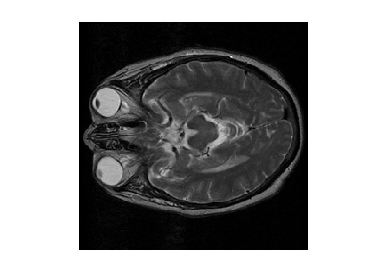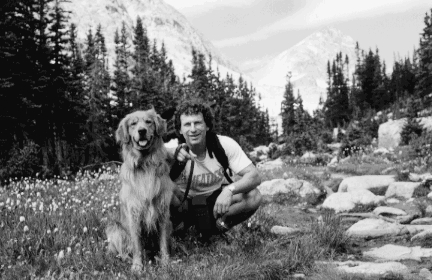## Introduction

To do image processing means to apply mathematical operations to an image for some specific purpose, e.g. make the edges sharper, remove noise (like bad TV reception), warp them (geometrically modify them in some fashion), compress them.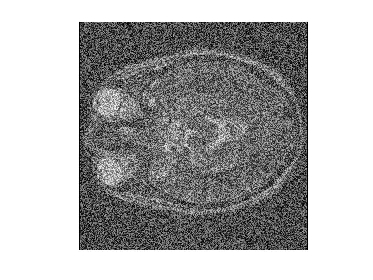Well, in order to apply mathematical operations to an image, we somehow have to interpret them as numbers. How? For simplicity, we'll assume that it's 1955 and there's only shades of grey to work with - no colour (so the scenery is rather dull ... like New Hampshire in winter).

## A Lighter shade of Pale

Let's begin with the simplest case: we have only black and white. We'll assign the number 0 to black, and 1 to white: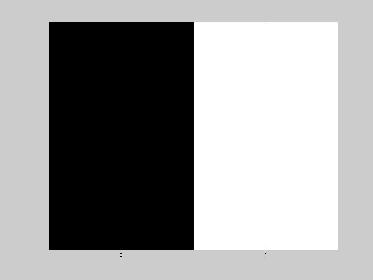Not very exciting perhaps, but it's a start. If we use four numbers, we have four shades of gray: 0 -> black, 1 -> a little lighter, 2 -> a little lighter still, 3 -> all white: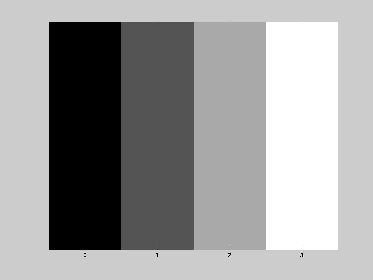And we can go further, using 8, or 16 and as much as 256 shades of gray: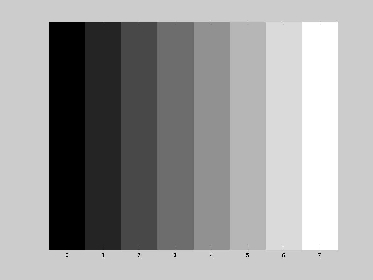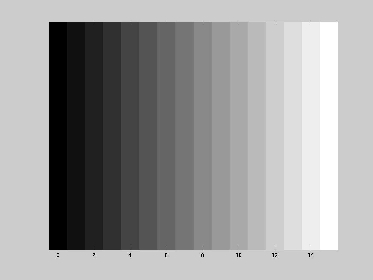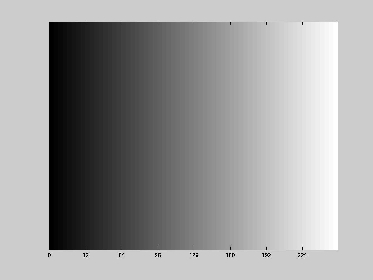By the time we reach 256 shades of gray, things look nice and smooth. Unlike the earlier cases, at this point we can barely see the differences between successive shades of gray.

Having assigned numbers to shades of gray, where's the image come in? It comes in this way: we consider an image to be an array (either square or rectangular) of numbers. Such an array of such numbers is called a matrix. For example, here's an array of four numbers, arranged in a square. Since this square has two rows and two columns, we call it a 2-by-2 matrix.

 0 10 10 20
If we draw a square with four boxes (2 per row, 2 per column), and give that square a shade of gray determined by its corresponding number (0 for pure black, 20 for pure white), the 2-by-2 matrix looks like this: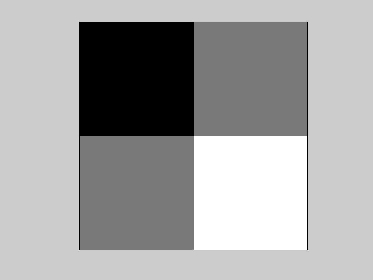Each box is called a pixel. Now let me make a square that has the same area as the one above, but this time has a total of 16 pixels, 4 per row, 4 per column, and assign a random shade of gray to each of those. The individual pixels get smaller: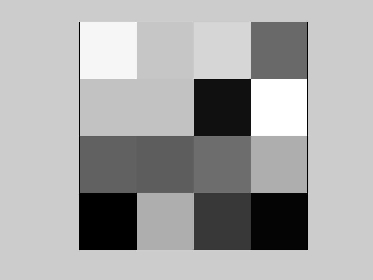Why stop there? Again, let me make a square that has the same area as the one above, but this time has a total of 64 pixels, 8 per row, 8 per column, and assign a random shade of gray to each of those. As before, the individual pixels get smaller: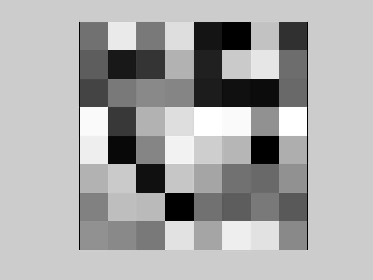Continuing this process, we'll eventually get to the point where the individual pixels are, for all practical purposes, invisible, and we see the image: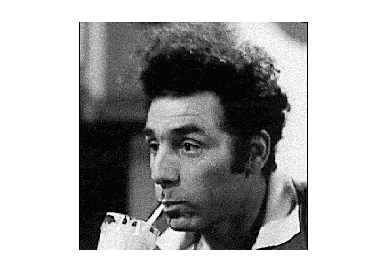The above image is a 256-by-256 (256 x 256) matrix. It has over 65,000 pixels.

Let's introduce a little mathematical notation. An image is a function f(x,y) that gives the level of brightness, or intensity at a specific spatial location (x,y). A shortcoming of computers is that they only work with digital data. So, to work with an image, we need to sample the function lots of times. This is why we consider images to be matrices (and vice versa). When we do something like remove noise from an image, all we're doing is applying some mathematical operations to a matrix whose entries are the pixel intensities.

To give just a brief hint of things to come ... how might we even begin to think about compressing Kramer? Suppose the above image is too large to fit on a single floppy disk. How might we be able to shrink the image? To store it on the floppy disk? One way to tackle this problem is to divide the matrix into pieces, and treat each piece as a separate, smaller problem: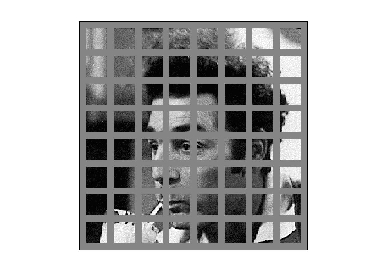This is the philosophy behind the jpeg format. We'll look into this later.

Abode Photoshop is a very powerful tool that allows one to do all sort of manipulations on images.

A Clean Sine Wave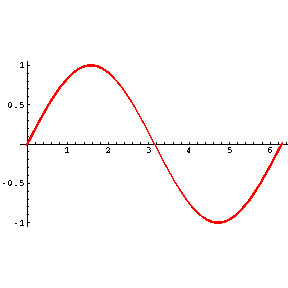A Noisy Sine Wave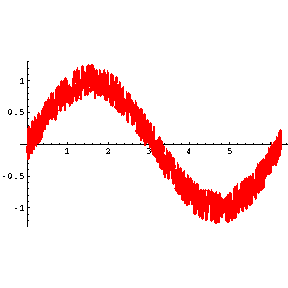## Homework

1. Fiddle around with Photoshop. Use some of the images below (or get your own from elsewhere) and apply some of the filters to get weird effects, like swirls or shears, or change colors. You are limited only by Photoshop and your imagination.
2. Here are two important image processing filters:
 -1 -1 -1 2 2 2 -1 -1 -1

 -1 2 -1 -1 2 -1 -1 2 -1

Use the two filters in Photoshop and try to determine how each effects an image. Do any particular features in the image become more (or less) apparent? What if the 2's were on one diagonal or the other? (If it isn't clear what's going on, try the filters on the image of LAX below.)
3. In Photoshop, combine two (or more) pictures into a single image, i.e. make a collage.

## Some random images

Los Angeles International Airport (LAX)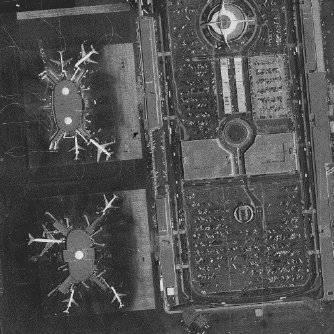A Brain